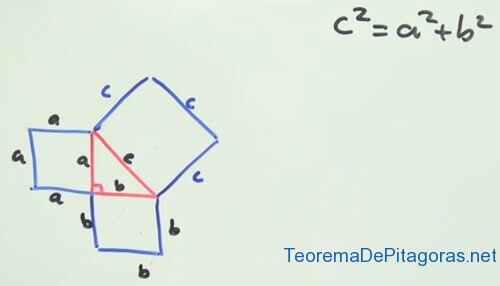# Proof of the Pythagorean Theorem (Video)

TOPICS: Squares              Theorem Proofs Exercises          Test       Graphic Representation

Next we’ll look at the proof of the Pythagorean Theorem. Although the equation that this theorem is known for is very popular a2 + b2 = C2 where C is the hypotenuse and a and b are the legs, the proof of this equation is not as well-known, but we will show you that it is really simple to prove the Pythagorean Theorem. To do this, we’re going to use a video which you can see at the end of the text, but first we recommend reading the explanation that we will give below.

Pythagoras realized that, in a right triangle, there was one side, opposite the 90 degree angle, that was longer than the other two. He called this side the hypotenuse and called the other two sides legs. He thought there must be a relationship between the measurements of the three sides of a right triangle and he researched it until he discovered that, if he made three squares, one from each of the sides of the triangle, the area of the largest square (which had the hypotenuse as one of its sides) was equal to the sum of the areas of the squares made by the smaller sides (the ones which had the legs as sides).This discovery gave rise to the Pythagorean theorem and this is the way we should use to prove the theorem if we are asked to in a problem or on a test. In the video we posted below, it will be very clear how to do a Proof of the Pythagorean Theorem using geometry and the squares that its sides form.